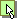DownloadcopyrightChangesPaper (PDF)

Download | Support
Expokit is a software package that provides matrix exponential routines for small dense or very large sparse matrices, real or complex. Here you will find the source code in Fortran and Matlab. The native Fortran version is embeddable in C/C++.

## Understanding Expokit

1. w(t) = exp(tA)v is the analytic solution of the homogeneous ODE problem: w'(t) = Aw(t), w(0) = v.

2. w(t) = exp(tA)v + t*phi(tA)u, where phi(x) = (exp(x)-1)/x, is the analytic solution of the nonhomogeneous ODE problem: w'(t) = Aw(t) + u, w(0) = v.
Expokit handles both cases.
Frequently Asked Questions

 From Computing Reviews: "This amazingly complete paper gives the theoretical background of the methods, presents applications, provides computational results for a standard set of pathological matrices, and closes with a mini-user's manual (...) It is entirely self-contained (...) Here we are given several sure ways to compute (...) exponentials, and these with rather sharp error bounds." [ Full Review ] Moler and Van Loan: "The most extensive software for computing the matrix exponential that we are aware of is Expokit." [ Nineteen Dubious Ways to Compute the Exponential of a Matrix, Twenty-Five Years Later. SIAM Review, Vol 45, No 1, March 2003. ]

Supported  by: